### 3D Quantum Hall Effect verified by SUSTech researchers

2019-04-10

Recently, Southern University of Science and Technology (SUSTech)Professor of Physics Lu Haizhouwas invited to write a particular perspective for National Science Review to introduce the new mechanism of this three-dimensional (3D) quantum Hall effect.

Professor Lu Haizhou worked with Academician Xie Xincheng of the Center for Quantum Materials from Peking University in writing a paper that showed a new mechanism for predicting the 3D quantum Hall effect. Physical Review Letters (PRL) published their paper. Renowned academic journal Nature then published the experimental progress of Fudan University XiuFaxian’s research group, which validated their new approach. Professor Lu Haizhou was a contributing author.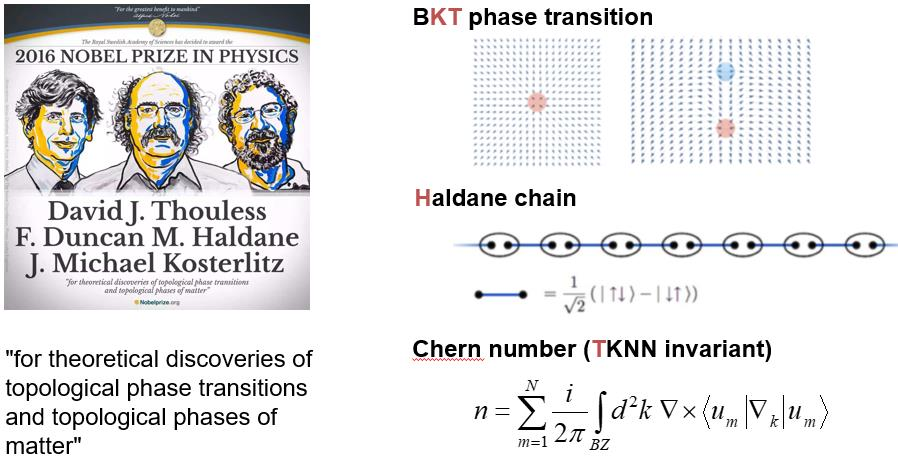(Figure 1. 2016 Nobel Prize in Physics-"topological phase transition and topological phases of matter. ")

In 2016, researchers of the topological phase transition and topological phases of matter received the Nobel Prize in Physics. Part of the reason for winning the Nobel Prize was that the researchers had discovered topological numbers on the "Quantum Hall Effect" which was found as part of the 1985 Nobel Prize in Physics.

Hall effect and quantum Hall effect

The Hall effects is a phenomenon in which electrons feel the Lorentz force perpendicular to the direction of a magnetic field and the direction  of motionthen deflecting and forming potential difference at both ends (shown in Figure 2).The ratio of the transverse voltage to the longitudinal current called Hall resistance, because the magnetic field causes the Hall effect.

In 1980, von Klitzing found that under the action of a strong magnetic field in a two-dimensional electron gas, Hall resistance quantization increases by integer levels, while the longitudinal resistance disappears. (as shown in Figure 2).

The discovery of the quantum Hall effect opens the door for the topology to enter the study of condensed matter physics. There have now been three Nobel Prizes in physics that relate to the quantum Hall effect (1985, 1998 and 2016).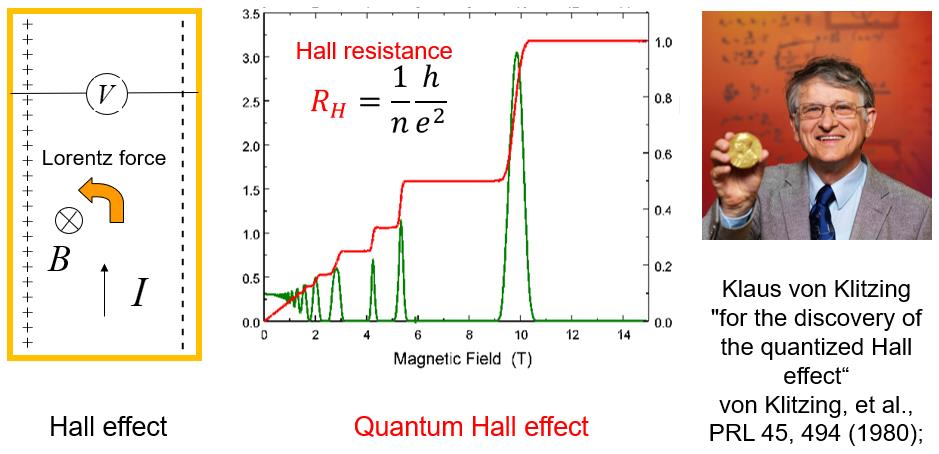Fig. 2 Hall Effect (left) and quantum Hall effect (medium)

Why would there be a quantized Hall effect?

Under a strong magnetic field, two-dimensional electron gas is quantized into a discrete Landau level (as shown in Figure 3, left). When the Fermi energy is between the two Landau level, the Landau level will produce a one-dimensional electric channel, called the edge states.  Each edge state can transport electrons without dissipation, and produce conductivity of e2/h size, because the magnetic field has limited the electrical conduction of the edge states, and there is no backscattering. The non-dissipative edge-state leads to the quantization of the Hall resistance and the disappearing longitudinal resistance.

The non-dissipative edge state in the quantum Hall effect is expected to be used in the future to achieve low energy consumption and high efficiency of electronic devices.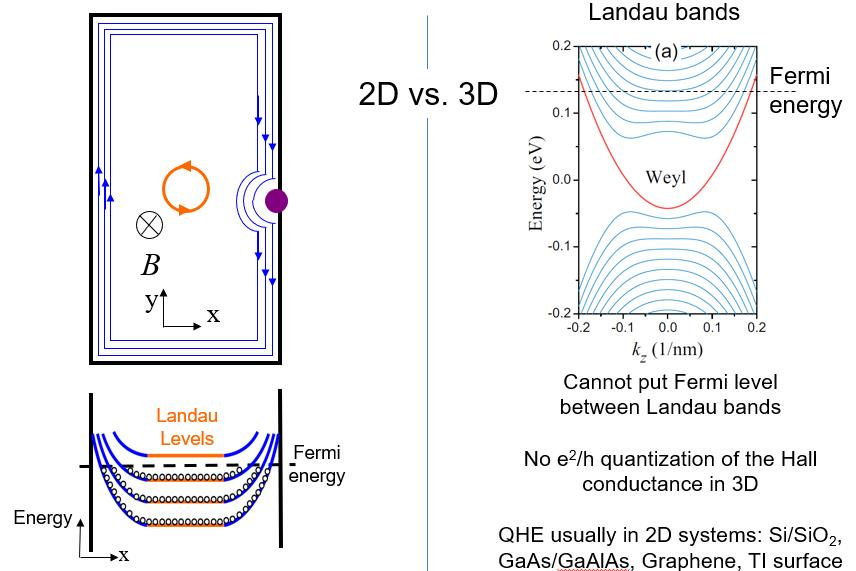Fig.3 Why there is a 2D quantum Hall effect (left) &3D are challenging to quantizatize(right)

From 2D to 3D

Since the discovery of the two-dimensional (2D) quantum Hall effect, theoretical work has begun to explore how to achieve quantization in a 3D system. In a 3D mode, electrons along the direction of the magnetic field do not feel the Lorentz force, and the momentum is still a good quantum number.  Landau energy level then becomes a dispersive Landau band in the direction of the magnetic field (as shown in Figure 3 on the right). The electronic transport of body carriers does not realize quantization. The quantum Hall effect is usually not observed in 3D systems, so if quantization can be found, it must be required to open the energy gap so that Fermi can be stay between the two Landau energy levels.

Early theories focused on how to open body energy gaps, such as the use of charge density waves or spin density waves, weak interlayer coupling and other mechanisms. Quantum Hall platforms conform to previously presented abstract images, including the latest nature articles contributed by Department of Physics Associate Professor Zhang Liyuan.

All the previous 3D quantum Hall effects focused on the quantization of Hall conductivity. Finding a new mechanism of 3D quantum Hall effect and realizing it in the experiment was still challenging.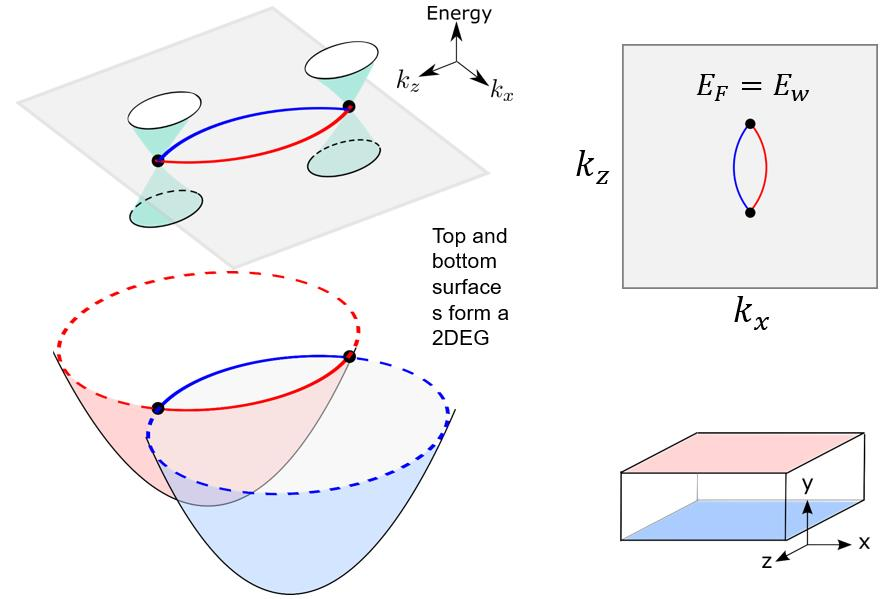Fig. 4 Topology Weyl semi-metallic Fermi arcs and Weyl points.

New 3D quantum Hall effect mechanism

Recently, Professor Lu Haizhou and Academician Xie Xincheng proposed a new three-dimensional quantum Hall effect mechanism. Topological semimetals are new members of the topological phase, with topologically protected surface states called Fermi arcs (as shown in Figure 4). In topological Weyl semi-metals, four surfaces can have topologically protected surface. Because of topological constraints, the surface state of each polygon is only half a 2D electron gas. The Fermi arc electron gas relative to the upper and lower surfaces can be connected by Weyl points to form a complete 2D electron gas. The strange phase was highly unexpected.

Since Fermi arcs is a 2D electron gas, can they have a quantum Hall effect? The first thing that needs to understand is what is the key to the formation of the Quantum Hall effect, which is the circumnutation of electrons (as shown in Figure 3, left). The quantum mechanical description of the electron cyclotron motion is equivalent to the harmonic oscillator, so the Landau energy levels of equal spacing are formed.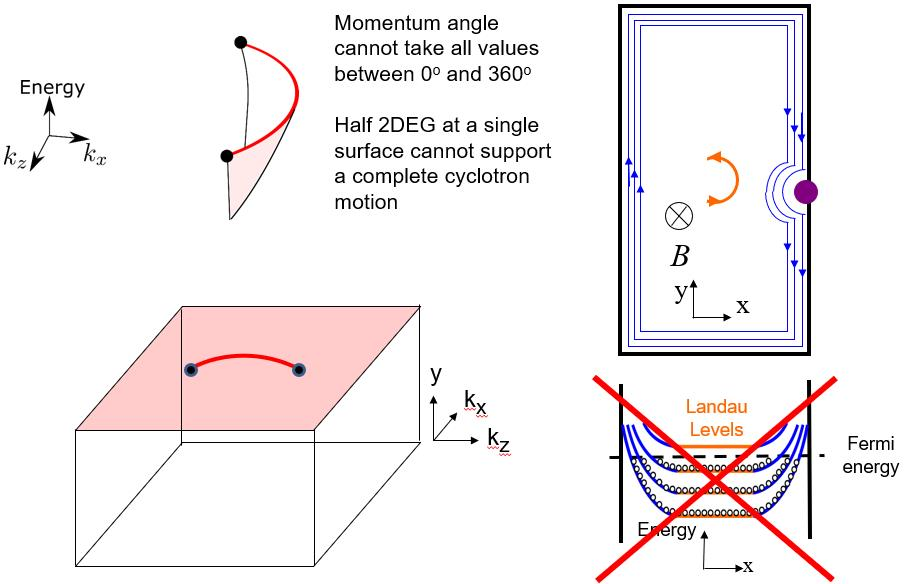Fig.5 A single surface of topological semimetals cannot support circumnutationand quantum Hall effect

On a single surface of the topological Weyl semimetal, each Fermi arc cannot take all the momentum angles. It means that the electrons cannot complete a complete circumnutation in real space when the magnetic field is driven by the Fermi arc so that a single surface of the topological semimetal cannot support the quantum Hall effect.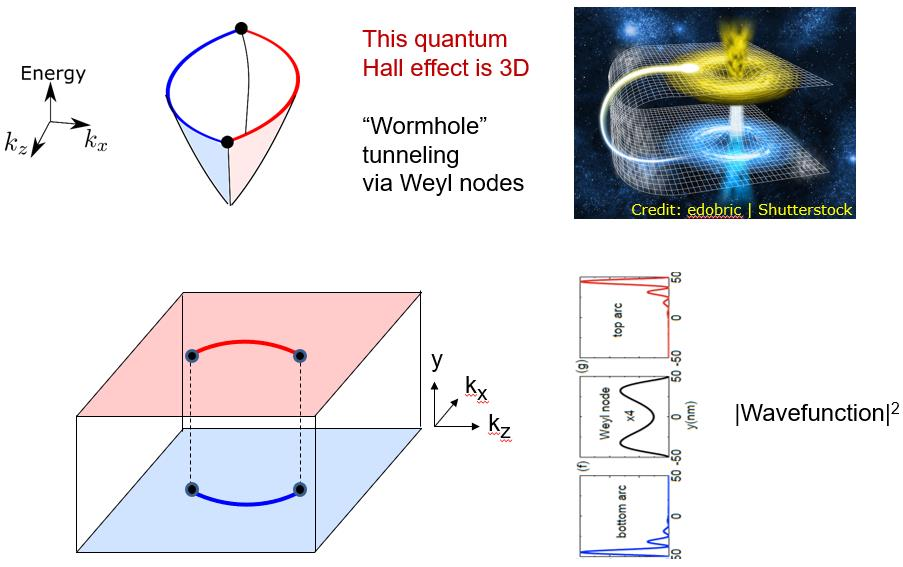Fig.6 The Fermi arc of the top and bottom surface of the topological Weyl semimetal through the Weyl point "wormhole" can support complete circumnutation and quantum Hall effect

The missing half of thecircumnutation, the "wormhole" tunnel achieve

The Fermi arc electron gas of the topological Weyl semimetal can form a complete electron gas. Electrons can perform a half circumnutation on the Fermi arc of a single surface and completes the other half of the circumnutation through the Weyl point in the semimetal (as shown in Figure 6). The Weyl point is the point of space contact between the conduction band and the valence band in the semi-metallic energy spectrum. The tunnel is similar to the "wormhole" effect, that is, the low-dimensional space-time can be connected through higher dimensional spatiotemporal singularity points.

Two infinitely distant 2D platforms together form a complete 2D electron gas and then support the quantum Hall effect, which is a new mechanism of 3D quantum Hall effect.Fig.7 Numerical simulation of the QHE in the topological semimetal slab.

Numerical simulation

The results of a numerical simulation also confirmed the new three-dimensional quantum Hall effect mechanism. They calculated the QHE of the topological semimetal slab, which could no longer be considered a 2D system. When Fermi energy  is at the Weyl point, the results of numerical simulation appeared a quantized  plateaus for Hall conductance (as shown in Figure 7).In particular, quantization is Hall conductance, not  Hall conductivity.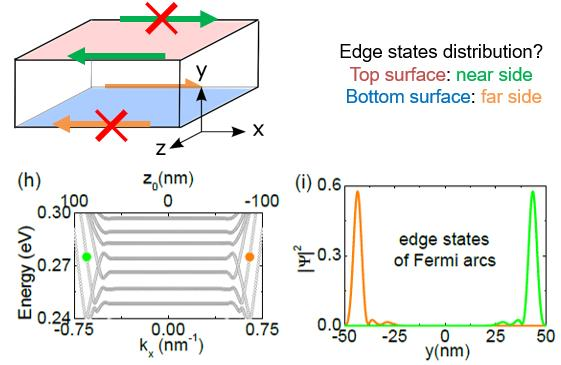Fig.8 The edge state of 3D quantum Hall effect, where the electrons only transmit along the "prism" of the sample.

The edge states of this 3D quantum Hall effect have a 3D distribution, on the near side (top surface) and the far side (bottom surface) (as shown in Figure 8). When the magnetic field reverses, so does the position of the edge state. The strange edge state distribution is thought to be detectable by various electron microscopy techniques in the future.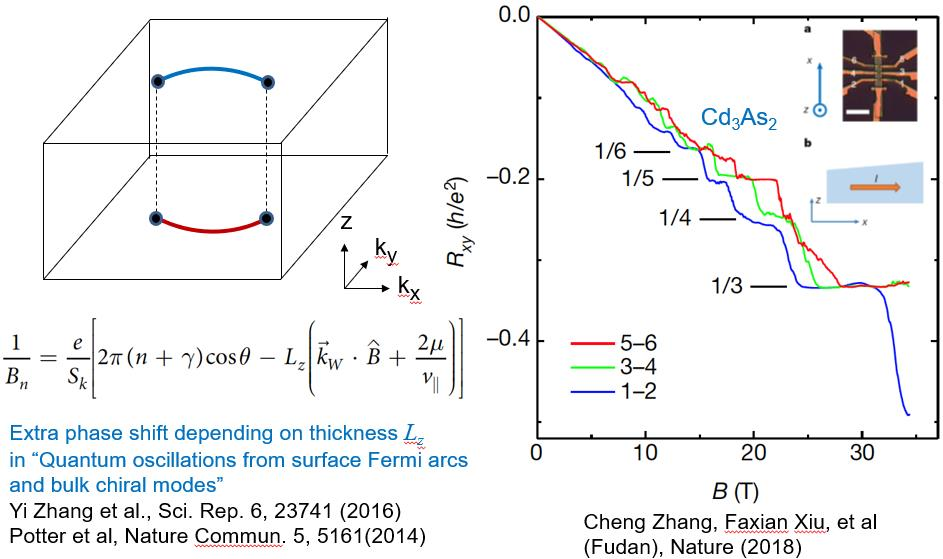Fig.9 3D quantum Hall effects have seen discovered in several experiments.

Progress of experiments

At present, several  experiments  have observed the quantization  plateaus of Hall resistance in topological semimetals (as shown in Figure 9). The study of this novel 3D quantum Hall effect has only just begun. The singular edge state distribution shown in Figure 8 will be a challenging direction for the future.

previous next：Progress in superconducting quantum computation at SUSTech: geometric quantum logic gates go faster and more robust next